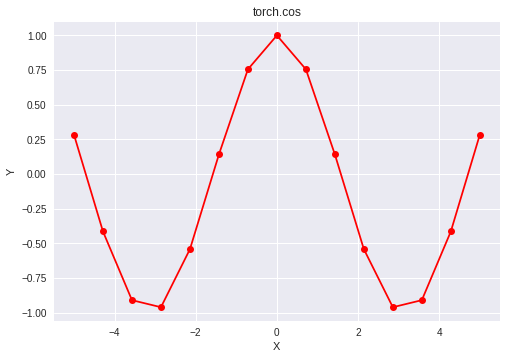# Python | PyTorch cos() method

• Last Updated : 12 Dec, 2021

PyTorch is an open-source machine learning library developed by Facebook. It is used for deep neural network and natural language processing purposes.
The function torch.cos() provides support for the cosine function in PyTorch. It expects the input in radian form and the output is in the range [-1, 1]. The input type is tensor and if the input contains more than one element, element-wise cosine is computed.

Syntax: torch.cos(x, out=None)
Parameters
x: Input tensor
name (optional): Output tensor
Return type: A tensor with the same type as that of x.

Code #1:

## Python3

 `# Importing the PyTorch library``import` `torch` `# A constant tensor of size 6``a ``=` `torch.FloatTensor([``1.0``, ``-``0.5``, ``3.4``, ``-``2.1``, ``0.0``, ``-``6.5``])``print``(a)` `# Applying the cos function and``# storing the result in 'b'``b ``=` `torch.cos(a)``print``(b)`

Output:

``` 1.0000
-0.5000
3.4000
-2.1000
0.0000
-6.5000
[torch.FloatTensor of size 6]

0.5403
0.8776
-0.9668
-0.5048
1.0000
0.9766
[torch.FloatTensor of size 6]```

Code #2: Visualization

## Python3

 `# Importing the PyTorch library``import` `torch` `# Importing the NumPy library``import` `numpy as np` `# Importing the matplotlib.pyplot function``import` `matplotlib.pyplot as plt` `# A vector of size 15 with values from -5 to 5``a ``=` `np.linspace(``-``5``, ``5``, ``15``)` `# Applying the cosine function and``# storing the result in 'b'``b ``=` `torch.cos(torch.FloatTensor(a))` `print``(b)` `# Plotting``plt.plot(a, b.numpy(), color ``=` `'red'``, marker ``=` `"o")``plt.title("torch.cos")``plt.xlabel("X")``plt.ylabel("Y")` `plt.show()`

Output:

``` 0.2837
-0.4138
-0.9090
-0.9598
-0.5414
0.1417
0.7556
1.0000
0.7556
0.1417
-0.5414
-0.9598
-0.9090
-0.4138
0.2837
[torch.FloatTensor of size 15]```My Personal Notes arrow_drop_up